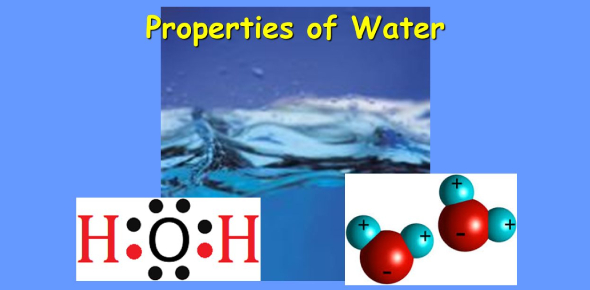# Properties Of Water Quiz: Trivia Test

10 Questions | Total Attempts: 26SettingsToday in this quiz, we are going to focus on the properties of water. Water is said to have less dense as a solid than as a liquid and has cohesive and adhesive properties. Did you know that water can conduct heat more quickly than any liquid except mercury? Do you think you understand how water would react when introduced to different properties? This quiz will help shed some light on that. Give it a try!

• 1.
According to the chart which has the greatest solubility in water?
• A.

Sand

• B.

Paper

• C.

Sugar

• 2.
Which is NOT BUOYANT in the water?
• A.

A penny

• B.

A stick

• C.

A raft

• 3.
The matter is made up of small bits called
• A.

Particles

• B.

Volume

• C.

Chunks

• 4.
A material that is less dense than water and will float is
• A.

Vinegar

• B.

Maple syrup

• C.

Oil

• 5.
What is the ability of matter to float in a liquid or a gas?
• A.

Buoyancy

• B.

Density

• C.

Volume

• 6.
A measure of the amount of material that will dissolve in another material is
• A.

Buoyancy

• B.

Solubility

• C.

Density

• 7.
A solid object that is denser than water will
• A.

Float in water

• B.

Sink in water

• C.

Make a solution

• 8.
Why does a helium-filled balloon float if you let go of the string?
• A.

Air is less dense than helium

• B.

The ballon is less dense than air

• C.

Helium is less dense than air

• 9.
What happens when submarine tanks are partly filled with water?
• A.

It helps it float to the surface

• B.

It helps it sink below the surface

• C.

It makes it sink to the very bottom of the water

• 10.
Any material that floats on water is
• A.

The same density as water

• B.Back to top